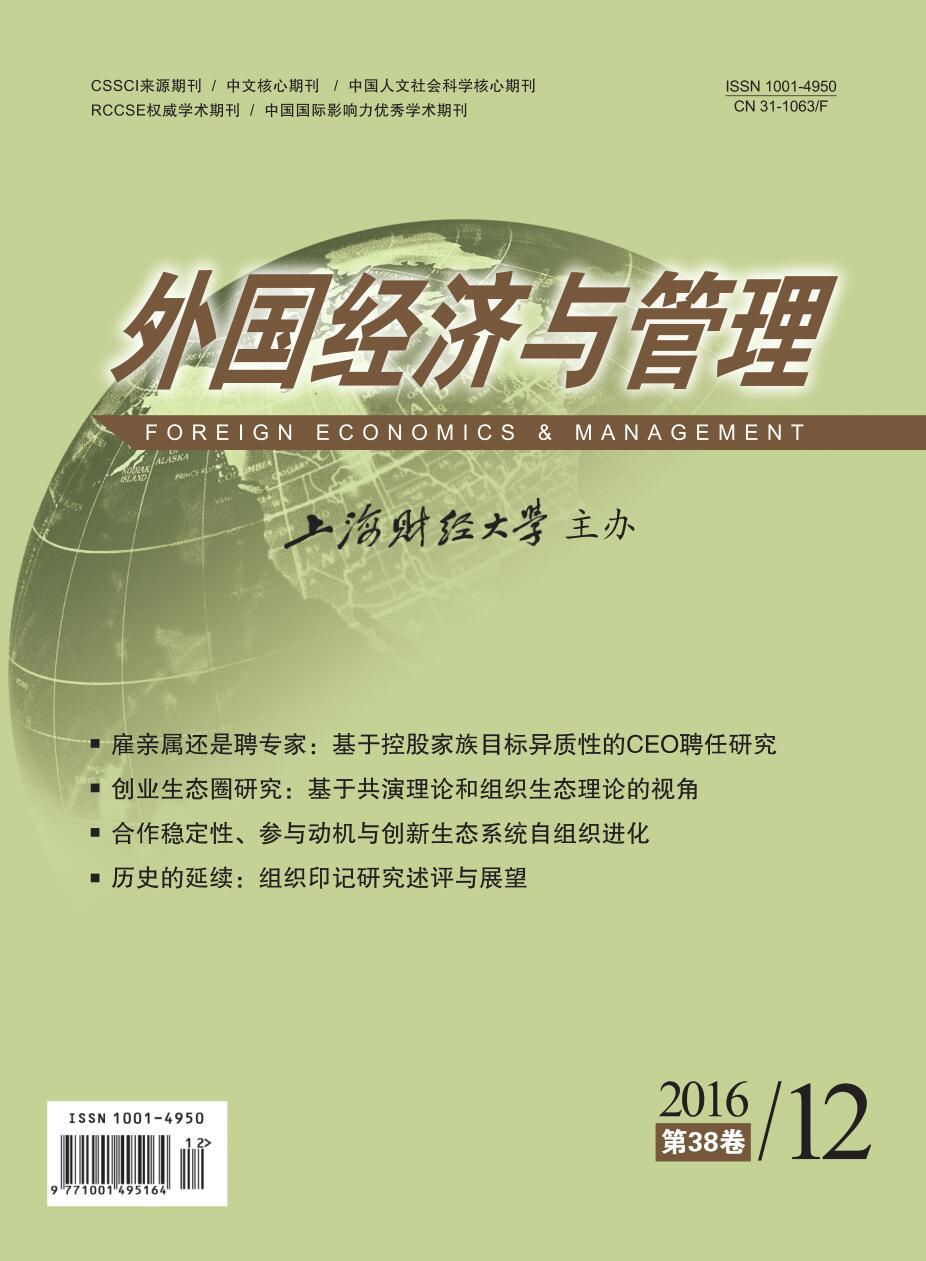﻿ 雇亲属还是聘专家:基于控股家族目标异质性的CEO聘任研究
 外国经济与管理2016, Vol. 38Issue (12): 3-180

#### 文章信息外国经济与管理2016年38卷第12期

Zhu Jian'an, Chen Ling, Wu Bingde

Hiring a Family CEO or a Non-family CEO? Research on the CEO Successor Choice Based on Heterogeneous Goals of Controlling Families

Foreign Economics & Management, 2016, 38(12): 3-18.

### 文章历史《外国经济与管理》
2016第38卷第12期

1.浙江大学 城市学院，浙江 杭州 310015;
2.浙江大学 管理学院，浙江 杭州 310058;
3.福州大学 经济与管理学院，福建 福州 350116;
4.浙江大学 企业家学院，浙江 杭州 310058

Hiring a Family CEO or a Non-family CEO? Research on the CEO Successor Choice Based on Heterogeneous Goals of Controlling Families
Zhu Jian'an1,4, Chen Ling2,4, Wu Bingde31.City College, Zhejiang University, Hangzhou 310015, China;
2.School of Management, Zhejiang University, Hangzhou 310058, China;
3.School of Economics & Management, Fuzhou University, Fuzhou 350116, China;
4.Institute of Entrepreneurs, Zhejiang University, Hangzhou 310058, China
Abstract: It is an important strategic succession decision to hire CEOs from family members or professional managers for the first generation entrepreneurs to handle over the management rights. A lot of studies on family business imply that CEOs who are members of the founding families underperform, but still a few family firms, even some public firms, hire the family members as CEOs in the reality. To explain the seemingly inefficiency succession decisions, this paper sets management professionalization of private firms under the background of trans-generation succession, extends the focus from profit-seeking firms to families behind the firms, and explores the antecedents of succession decisions from the perspectives of the heterogeneous goals of controlling families. According to a multi-task principal-agent model, it establishes a matching framework between companies and CEOs driven by noneconomic goals of controlling families, and finds that families that pay more attention to noneconomic goals tend to give lower-level incentive compensation contracts, require lower-degree capabilities of the CEO candidates to run the businesses and prefer the family identity of CEOs. It tries to confirm that the succession decisions of CEOs are endogenous from the heterogeneous goals of controlling families, and make up the theoretical gap of logic relationship between willingness and abilities of families. The analysis of the antecedents of succession decisions of family firms deepens the organization theory in the context of family businesses and gives some advices on succession decisions for the first generation entrepreneurs.
Key words: family business; CEO succession; heterogeneous goal; willingness and ability; matching

(一) 雇亲属还是聘专家：有何不同？

(二) 家族企业职业化研究可能的不足

(一) 多任务目标下的家族企业CEO薪酬激励强度决定模型

 ${\left[{\theta V_1^\rho + \left( {1 - \theta } \right)V_2^\rho } \right]^{\frac{1}{\rho }}}$

 ${{V}_{1}}={{e}_{1}}+{{\varepsilon }_{1}},\ {{V}_{2}}={{e}_{2}}+{{\varepsilon }_{2}},\ {{\varepsilon }_{i}}\tilde{\ }N\left( 0,\sigma _{i}^{2} \right),\ {{\sigma }_{12}}={{\sigma }_{21}}=0$

CEO的薪酬取决于两项任务的完成情况，并从中分别获得β1β2的分成比例，薪酬函数表达式为：

 $W\left( {{V_1},{V_2}} \right) = \alpha + {\beta _1}{V_1} + {\beta _2}{V_2}$

 \begin{align} & \text{UCE=}\alpha +{{\beta }_{1}}{{e}_{1}}+{{\beta }_{2}}{{e}_{2}}-\frac{1}{2}e_{1}^{2}-\frac{1}{2}e_{2}^{2} \\ & -\frac{1}{2}r\sigma _{1}^{2}\beta _{1}^{2}-\frac{1}{2}r\sigma _{2}^{2}\beta _{2}^{2} \\ \end{align}

 $\frac{\beta _{1}^{*}}{\beta _{2}^{*}}={{\left[\frac{\theta }{1-\theta }\cdot \frac{1+r\sigma _{2}^{2}}{1+r\sigma _{1}^{2}} \right]}^{\frac{1}{2-\rho }}}$

(二) 家族企业的非经济目标与CEO能力的匹配模型

 $\theta {{V}} + \left( {1 - \theta } \right)\left( {{{S}} - \lambda \beta } \right)$

 ${{V}} = {\lambda ^{\frac{1}{2}}}\left( {e + \varepsilon } \right),\;\varepsilon \sim N\left( {0,{\sigma ^2}} \right)$

CEO的效用函数为CARA函数：

 $U\left( \varpi \right) = - {{\rm{e}}^{ - R\varpi }}$

 ${{UCE = }}\alpha + \beta {\lambda ^{\frac{1}{2}}}e - \frac{1}{2}{e^2} - \frac{1}{2}r\lambda {\beta ^2}\sigma$

 $\begin{array}{l} E\pi = \left[{\theta V + \left( {1 - \theta } \right)\left( {{\rm{S}} - \lambda \beta } \right)} \right] - W\left( {{V}} \right)\\ \theta V + \left( {1 - \theta } \right)\left( {{\rm{S}} - \lambda \beta } \right) - \alpha - \beta V\\ s.t.\;\max UC{E_{\left( {{\rm{IC}}} \right)}}\\ \;\;\;\;\;UCE \geqslant {\underline U _{\left( {{\rm{IR}}} \right)}} \end{array}$

 ${{\beta }^{*}}=\frac{2\theta -1}{1+r{{\sigma }^{2}}}$ (4)

TS表示社会总剩余，由公司的预期收益与CEO的预期效用函数共同组成：

 \begin{align} & TS=E\pi +UCE=\theta \beta \lambda +\left( 1-\theta \right)S- \\ & \left( 1-\theta \right)\lambda \beta +\underset{\raise0.3em\hbox{\smash{\scriptscriptstyle-}}}{U}-\frac{1}{2}{{\beta }^{2}}\lambda -\frac{1}{2}r{{\beta }^{2}}\lambda {{\sigma }^{2}} \\ \end{align} (5)

 $TS = \left( {1 - \theta } \right){{S}} + \frac{{{{\left( {2\theta - 1} \right)}^2}\lambda }}{{2\left( {1 + {{r}} {\sigma ^2}} \right)}}$

 $\begin{array}{*{35}{l}} \left( 1-{{\theta }_{A}} \right)S+\frac{{{\left( 2{{\theta }_{A}}-1 \right)}^{2}}{{\lambda }_{1}}}{2\left( 1+r{{\sigma }^{2}} \right)}+\left( 1-{{\theta }_{B}} \right)S+\frac{{{\left( 2{{\theta }_{B}}-1 \right)}^{2}}{{\lambda }_{2}}}{2\left( 1+r{{\sigma }^{2}} \right)} \\ \left( 1-{{\theta }_{A}} \right)S+\frac{{{\left( 2{{\theta }_{A}}-1 \right)}^{2}}{{\lambda }_{2}}}{2\left( 1+r{{\sigma }^{2}} \right)}+\left( 1-{{\theta }_{B}} \right)S+\frac{{{\left( 2{{\theta }_{B}}-1 \right)}^{2}}{{\lambda }_{1}}}{2\left( 1+r{{\sigma }^{2}} \right)} \\ \end{array}$

 $\left[{{{\left( {2{\theta _A} - 1} \right)}^2} - {{\left( {2{\theta _B} - 1} \right)}^2}} \right] {\lambda _1} \geqslant \left[{{{\left( {2{\theta _A} - 1} \right)}^2} - {{\left( {2{\theta _B} - 1} \right)}^2}} \right] {\lambda _2}$

θAθBλ1λ2条件下，这个不等式显然是成立的。

(三) 控股家族异质性目标下的CEO聘任

 身份 所需能力 激励强度 聘任决策 重视经济目标 无差异（家族/职业经理人） 高（职业经理人） 大（职业经理人） 职业经理人 重视非经济目标 重要（家族成员） 低（家族成员） 小（家族成员） 家族成员图 1 基于家族目标异质性的CEO聘任决策